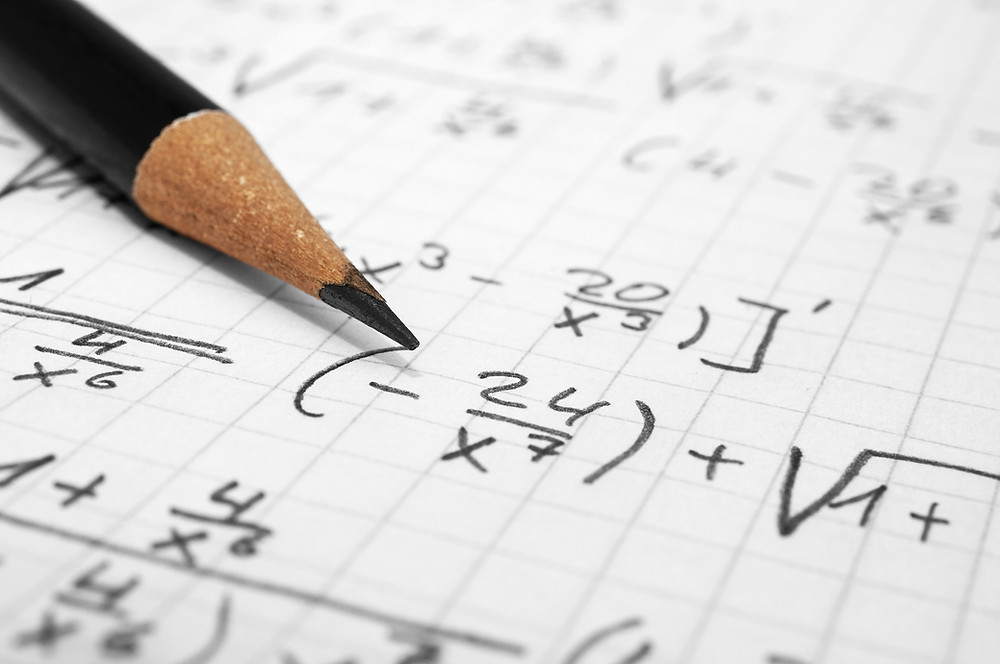Search

# The Importance of Mathematics in the Development of Science

Mathematics is widely used in Science to calculate the measurements of objects, draw conclusions from raw data and to learn the relationship between different functions and properties.Mathematics can be used to describe data Descriptive statistics are used to describe, represent and understand the features of a specific data set by giving short summaries about the sample and measures of the data set. Descriptive statistics include numbers, tables, charts, and graphs. Measures of the center The mean refers to the average of a given data set. You can easily determine the mean by doing a very simple calculation! Add up all the numbers, then divide by how many numbers there are.

For example, the mean of 11,12 and 13 is (11+12+13)/3 =12

The median refers to the middle point in a data set when it is ordered from least to greatest. The median is also the number that is halfway into the set. In the case where a given data set contains an even number of values, the median will be equal to the average of the two values in the middle.

For example, the median of 10,11,12,13 is (11+12)/2 = 11.5

The mode refers to the most commonly observed value in a data set.